Courses

# Spring - MCQ Test 1

## 20 Questions MCQ Test Mock Test Series - Mechanical Engineering (ME) for GATE 2020 | Spring - MCQ Test 1

Description
This mock test of Spring - MCQ Test 1 for Mechanical Engineering helps you for every Mechanical Engineering entrance exam. This contains 20 Multiple Choice Questions for Mechanical Engineering Spring - MCQ Test 1 (mcq) to study with solutions a complete question bank. The solved questions answers in this Spring - MCQ Test 1 quiz give you a good mix of easy questions and tough questions. Mechanical Engineering students definitely take this Spring - MCQ Test 1 exercise for a better result in the exam. You can find other Spring - MCQ Test 1 extra questions, long questions & short questions for Mechanical Engineering on EduRev as well by searching above.
QUESTION: 1

### If the wire diameter of a closed coil helical spring subjected to compressive load is increased from 1 cm to 2 cm, other parameters remaining same, then deflection will decrease by a factor of:

Solution: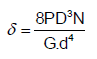QUESTION: 2

### Two helical tensile springs of the same material and also having identical mean coil diameter and weight, have wire diameters d and d/2. The ratio of their stiffness is:

Solution: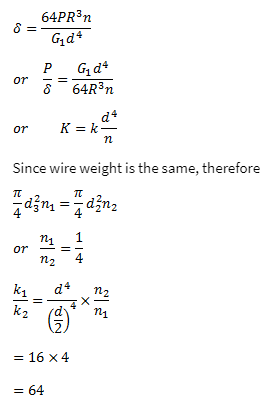QUESTION: 3

### A weighing machine consists of a 2 kg pan resting on spring. In this condition, with the pan resting on the spring, the length of the spring is 200 mm. When a mass of 20 kg is placed on the pan, the length of the spring becomes 100 mm. For the spring, the undeformed length lo and the spring constant k (stiffness) are: [GATE-2005]

Solution:

Initial length = lo m and stiffness = k N/m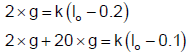Just solve the above equations.

QUESTION: 4

A helical coil spring with wire diameter ‟d‟ and coil diameter 'D' is subjected to external load. A constant ratio of d and D has to be maintained, such that the extension of spring is independent of d and D. What is this ratio?

Solution: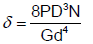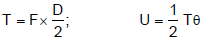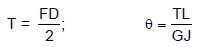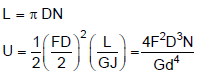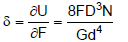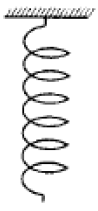QUESTION: 5

Assertion (A): Concentric cylindrical helical springs are used to have greater spring force in a limited space.

Reason (R): Concentric helical springs are wound in opposite directions to prevent locking of coils under heavy dynamic loading.

Solution:
QUESTION: 6

Which one of the following statements is correct?

If a helical spring is halved in length, its spring stiffness

Solution:

Stiffness of sprin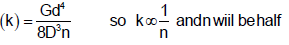QUESTION: 7

A close-coiled helical spring is made of 5 mm diameter wire coiled to 50 mm mean diameter. Maximum shear stress in the spring under the action of an axial force is 20 N/mm2. The maximum shear stress in a spring made of 3 mm diameter wire coiled to 30 mm mean diameter, under the action of the same force will be nearly

Solution: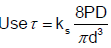QUESTION: 8

The maximum shear stress occurs on the outermost fibers of a circular shaft under torsion. In a close coiled helical spring, the maximum shear stress occurs on the

Solution:
QUESTION: 9

A closed-coil helical spring is subjected to a torque about its axis. The spring wire would experience a

Solution:
QUESTION: 10

A closely coiled helical spring of 20 cm mean diameter is having 25 coils of 2 cm diameter rod. The modulus of rigidity of the material if 107 N/cm2. What is the stiffness for the spring in N/cm?

Solution:

Stiffness of sprin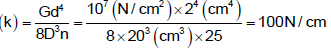QUESTION: 11

In the calculation of induced shear stress in helical springs, the Wahl's correction factor is used to take care of

Solution:
QUESTION: 12

Cracks in helical springs used in Railway carriages usually start on the inner side of the coil because of the fact that

Solution:
QUESTION: 13

A length of 10 mm diameter steel wire is coiled to a close coiled helical spring having 8 coils of 75 mm mean diameter, and the spring has a stiffness K. If the same length of wire is coiled to 10 coils of 60 mm mean diameter, then the spring stiffness will be:

Solution:

Stiffnessof spring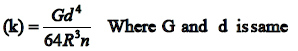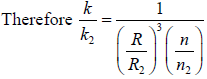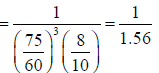QUESTION: 14

A spring with 25 active coils cannot be accommodated within a given space. Hence 5 coils of the spring are cut. What is the stiffness of the new spring?

Solution: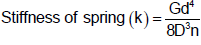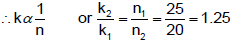QUESTION: 15

When two springs of equal lengths are arranged to form cluster springs which of the following statements are the:

1. Angle of twist in both the springs will be equal

2. Deflection of both the springs will be equal

3. Load taken by each spring will be half the total load

4. Shear stress in each spring will be equal

Solution:
QUESTION: 16

The unit of modulus of elasticity is same as those of

Solution:
QUESTION: 17

A helical spring of constant k is cut into four equal pieces and the four pieces are then combined in parallel. The equivalent spring constant will be

Solution:
QUESTION: 18

If a compression coil spring is cut into two equal parts and the parts are then used in parallel, the ratio of the spring rate to its initial value will be :

Solution:

When a spring is cut into two, no. of coils gets halved.
∴ Stiffness of each half gets doubled.
When these are connected in parallel, stiffness = 2k + 2k = 4k

QUESTION: 19

Two coiled springs, each having stiffness K, are placed in parallel. The stiffness of the combination will be:

Solution: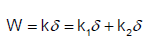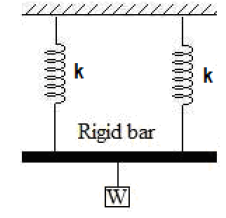QUESTION: 20

Figure given above shows a springmass system where the mass m is fixed in between two springs of stiffness S1 and S2. What is the equivalent spring stiffness?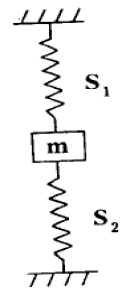Solution: